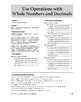# Math Fundamentals Unit: Use Operations with Whole Numbers and Decimals, Grade 5Subject
Resource Type
Product Rating
File Type

PDF (Acrobat) Document File

Be sure that you have an application to open this file type before downloading and/or purchasing.

7 MB|38 pages
Share
Product Description

This download contains a unit from Evan-Moor’s Math Fundamentals and includes: a teacher unit overview page, reproducible student pages with math models and skill practice, and an answer key.

This unit practices the following math skills:

● Multiplying whole numbers

● Dividing whole numbers

● Adding and subtracting decimals

● Multiplying decimals

● Dividing decimals

Comprehensive but not complicated! Math Fundamentals helps your fifth grade students gain a conceptual understanding of math and develops their problem-solving skills with math models, “think” questions, plenty of skill practice, and real-world problems. Each unit guides students in thinking through, analyzing, and solving problems.

To help you target instruction, each unit clearly lists the standards information, mathematical practices, and skills covered.

Within a unit, math lessons are presented simply. Every math lesson includes:

A Math Models reference page that shows students strategies for solving problems. This is helpful for parents and students transitioning to new math.

Skill practice pages that progress in difficulty.

A culminating problem-solving activity that leads students through the process of solving a real-life problem.

Get the entire Math Fundamentals e-book for \$19.99:

Total Pages
38 pages